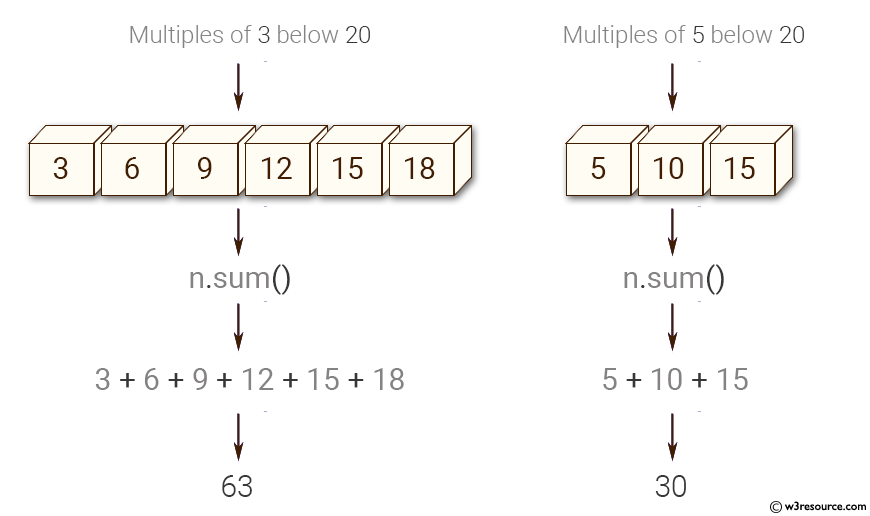﻿ NumPy: Sum of all the multiples of 3 or 5 below 100 - w3resource# NumPy: Sum of all the multiples of 3 or 5 below 100

## NumPy: Array Object Exercise-68 with Solution

Write a NumPy program (using numpy) to sum of all the multiples of 3 or 5 below 100.

Pictorial Presentation:Sample Solution:

Python Code:

``````import numpy as np
x = np.arange(1, 100)
# find  multiple of 3 or 5
n= x[(x % 3 == 0) | (x % 5 == 0)]
print(n[:1000])
# print sum the numbers
print(n.sum())
```
```

Sample Output:

```[ 3  5  6  9 10 12 15 18 20 21 24 25 27 30 33 35 36 39 40 42 45 48 50 5
1 54
55 57 60 63 65 66 69 70 72 75 78 80 81 84 85 87 90 93 95 96 99]
2318
```

Python Code Editor:

Have another way to solve this solution? Contribute your code (and comments) through Disqus.

What is the difficulty level of this exercise?

Test your Python skills with w3resource's quiz

﻿

## Python: Tips of the Day

List comprehension:

```>>> m = [x ** 2 for x in range(5)]
>>> m
[0, 1, 4, 9, 16]
```